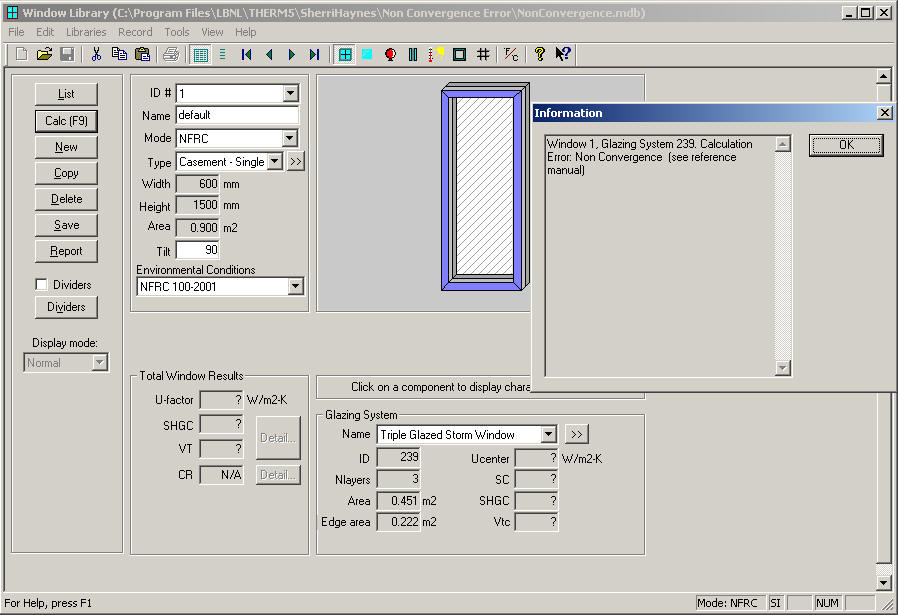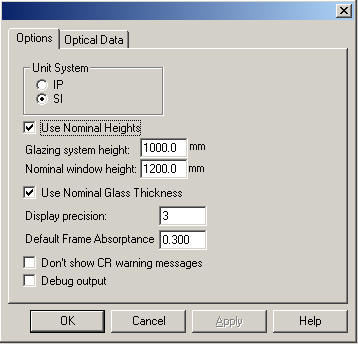# Glazing System Non-Convergence Error Message

Last Updated:
Tuesday, September 5, 2017

### Software/Versions Affected:

There are some circumstances where Berkeley Lab WINDOW 5 will give the following error message:This error can occur either in the Window or Glazing System calculation, but it is actually an error that occurs when the program tries to calculate the glazing system thermal properties — it occurs in the Window Library because the program recalculates the center-of-glass U-value based on the window height. It will happen in rare circumstances because of a problem with the discontinuity in correlations that calculate convective heat transfer in glazing cavities.

The solution is to change either the glazing system height or width. In general, the most practical solution is to change the glazing system height rather than the width..

WINDOW 5 uses a default glazing system height of 1000 mm when calculating the U-factor in the Glazing System Library. However, in the Window Library, the program will use the defined window height to re-calculate the glazing system U-factor to get the center-of-glass value to use in the whole-window calculation. If the program calculated the glazing system U-factor in the Glazing System Library without error, but then shows the "Non Convergence" error in the Window Library, the problem is that the glazing system height, now based on the window height relative to the glazing system width, creates the thermal condition that coincides with the discontinuity in the correlation.

The solution is to decrease the window height. This can be done in two ways:

1. Adjust the height in the Window Library Detailed screen
2. Change the value for "Nominal window height" in the File/Preferences/Options screen, and also Check the "Use Nominal Heights" checkbox. This will override the actual window height with the nominal height in the calculation.

Option 2 is the preferable choice, so that the other properties calculated for the whole window will be according to the actual window dimensions.

The process of determining what glazing system height is required for the program to perform the calculation without error is an iterative process. There will most likely be heights above and below the problem height that will allow the calculation to proceed. Once these heights are determined, do a whole-window calculation (in the Window Library) for each case, and compare the U-factors.

In general, the higher, more "punitive" U-factor should be selected, so that you are not getting U-factor "credit" for an artificially set glazing system height. The U-value differences between the cases should be relatively small.

## Example

The window below does not calculate, instead giving the "Non Convergence" error message.

The window is 1500 mm high, so the first thing to do is to determine at what heights the window will calculate.In the Preferences (File/Preferences/Options) dialog box, check the "Use Nominal Heights" checkbox, and change the "Nominal window height" field (maybe in increments of 100 mm), and then go back to the Window Library to see if the window will calculate at the new nominal window height. (Leave the Glazing system height defaulted to 1000 mm).For this example, the window would calculate at a Nominal window height of 1275 mm and also at 1925 mm, so these are the two heights that bound the U-factor results. Here are the U-factor results for each Nominal window height:

Nominal Window Height U-factor
1275 mm 0.713
1925 mm 0.711

In this case, the higher U-factor (the "punitive" case) is 0.713, which corresponds to the 1275 mm window height, so that is the U-factor that should be used as the result of this calculation.